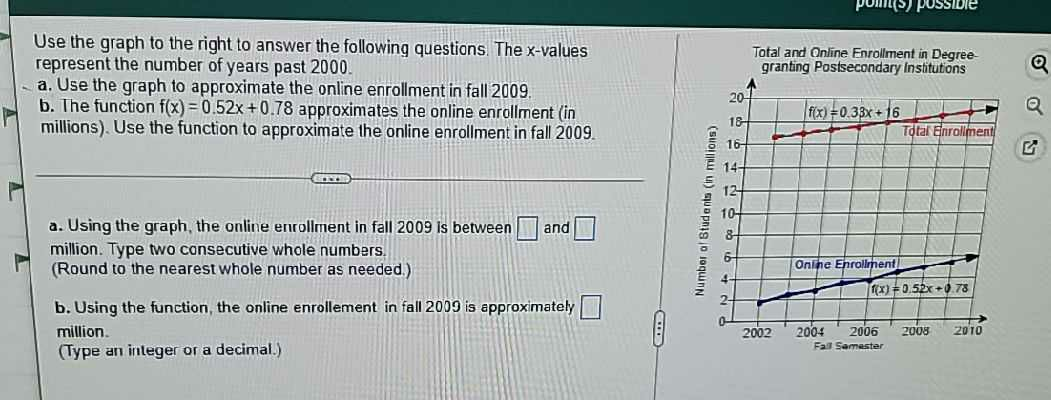### Still have math questions?

Algebra
QuestionUse the graph to the right to answer the following questions. The $$x$$ -values represent the number of years past $$2000$$ .

a. Use the graph to approximate the online enrollment in fall $$2009$$ .

b. The function $$f ( x ) = 0.52 x + 0.78$$ approximates the online enrollment (in millions). Use the function to approximate the online enrollment in fall $$2009$$ .

a. Using the graph, the online enrollment in fall $$2009$$ is between $$\square$$ and $$\square$$ million. Type two consecutive whole numbers.

(Round to the nearest whole number as needed.)

b. Using the function, the online enrollment in fall $$2009$$ is approximately $$\square$$ million.

(Type an integer or a decimal.)